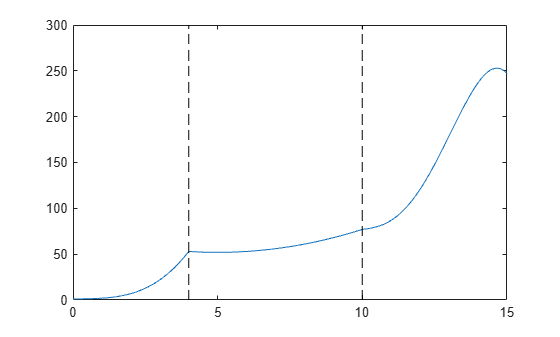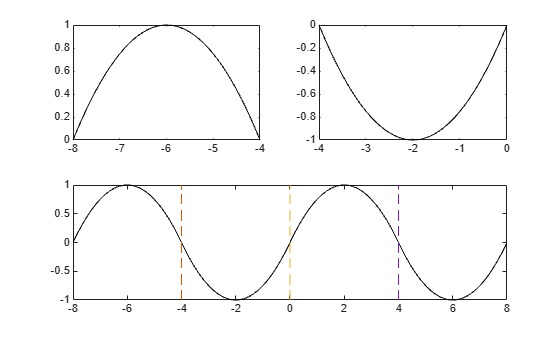# ppval

Evaluate piecewise polynomial

## Syntax

``v = ppval(pp,xq)``

## Description

example

````v = ppval(pp,xq)` evaluates the piecewise polynomial `pp` at the query points `xq`.```

## Examples

collapse all

Create a piecewise polynomial that has a cubic polynomial in the interval [0,4], a quadratic polynomial in the interval [4,10], and a quartic polynomial in the interval [10,15].

```breaks = [0 4 10 15]; coefs = [0 1 -1 1 1; 0 0 1 -2 53; -1 6 1 4 77]; pp = mkpp(breaks,coefs)```
```pp = struct with fields: form: 'pp' breaks: [0 4 10 15] coefs: [3x5 double] pieces: 3 order: 5 dim: 1 ```

Evaluate the piecewise polynomial at many points in the interval [0,15] and plot the results. Plot vertical dashed lines at the break points where the polynomials meet.

```xq = 0:0.01:15; plot(xq,ppval(pp,xq)) line([4 4],ylim,'LineStyle','--','Color','k') line([10 10],ylim,'LineStyle','--','Color','k')```Create and plot a piecewise polynomial with four intervals that alternate between two quadratic polynomials.

The first two subplots show a quadratic polynomial and its negation shifted to the intervals [-8,-4] and [-4,0]. The polynomial is

`$1-{\left(\frac{x}{2}-1\right)}^{2}=\frac{-{x}^{2}}{4}+x.$`

The third subplot shows a piecewise polynomial constructed by alternating these two quadratic pieces over four intervals. Vertical lines are added to show the points where the polynomials meet.

```subplot(2,2,1) cc = [-1/4 1 0]; pp1 = mkpp([-8 -4],cc); xx1 = -8:0.1:-4; plot(xx1,ppval(pp1,xx1),'k-') subplot(2,2,2) pp2 = mkpp([-4 0],-cc); xx2 = -4:0.1:0; plot(xx2,ppval(pp2,xx2),'k-') subplot(2,1,2) pp = mkpp([-8 -4 0 4 8],[cc;-cc;cc;-cc]); xx = -8:0.1:8; plot(xx,ppval(pp,xx),'k-') hold on line([-4 -4],ylim,'LineStyle','--') line([0 0],ylim,'LineStyle','--') line([4 4],ylim,'LineStyle','--') hold off```## Input Arguments

collapse all

Piecewise polynomial, specified as a structure. You can create `pp` using `spline`, `pchip`, `makima`, `interp1`, or the spline utility function `mkpp`.

Query points, specified as a vector or array. `xq` specifies the points where `ppval` evaluates the piecewise polynomial.

Data Types: `single` | `double`

## Output Arguments

collapse all

Piecewise polynomial values at query points, returned as a vector, matrix, or array.

If `pp` has `[d1,..,dr]`-valued coefficients (nonscalar coefficient values), then:

• When `xq` is a vector of length `N`, `v` has size `[d1,...,dr,N]`, and `v(:,...,:,j)` is the value at `xq(j)`.

• When `xq` has size `[N1,...,Ns]`, `v` has size `[d1,...,dr,N1,...,Ns]`, and ```v(:,...,:, j1,...,js)``` is the value at `xq(j1,...,js)`.

## Version History

Introduced before R2006a